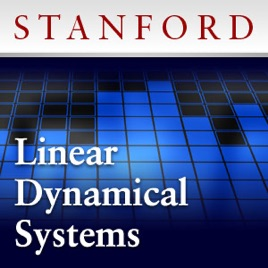20 episodes

Introduction to applied linear algebra and linear dynamical systems, with applications to circuits, signal processing, communications, and control systems.

Topics include: Least-squares aproximations of over-determined equations and least-norm solutions of underdetermined equations. Symmetric matrices, matrix norm and singular value decomposition. Eigenvalues, left and right eigenvectors, and dynamical interpretation. Matrix exponential, stability, and asymptotic behavior. Multi-input multi-output systems, impulse and step matrices; convolution and transfer matrix descriptions. Control, reachability, state transfer, and least-norm inputs. Observability and least-squares state estimation.Introduction to Linear Dynamical Systems Stanford

• Education

Introduction to applied linear algebra and linear dynamical systems, with applications to circuits, signal processing, communications, and control systems.

Topics include: Least-squares aproximations of over-determined equations and least-norm solutions of underdetermined equations. Symmetric matrices, matrix norm and singular value decomposition. Eigenvalues, left and right eigenvectors, and dynamical interpretation. Matrix exponential, stability, and asymptotic behavior. Multi-input multi-output systems, impulse and step matrices; convolution and transfer matrix descriptions. Control, reachability, state transfer, and least-norm inputs. Observability and least-squares state estimation.

3.9 out of 5
16 Ratings

16 Ratings